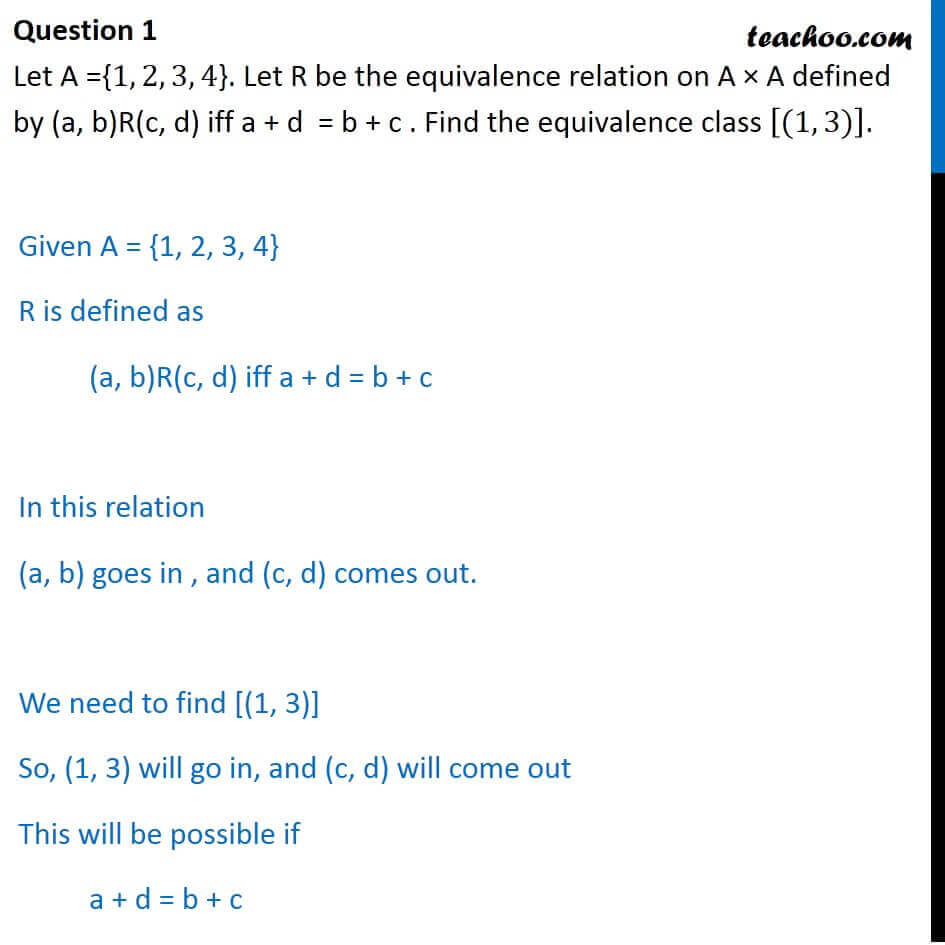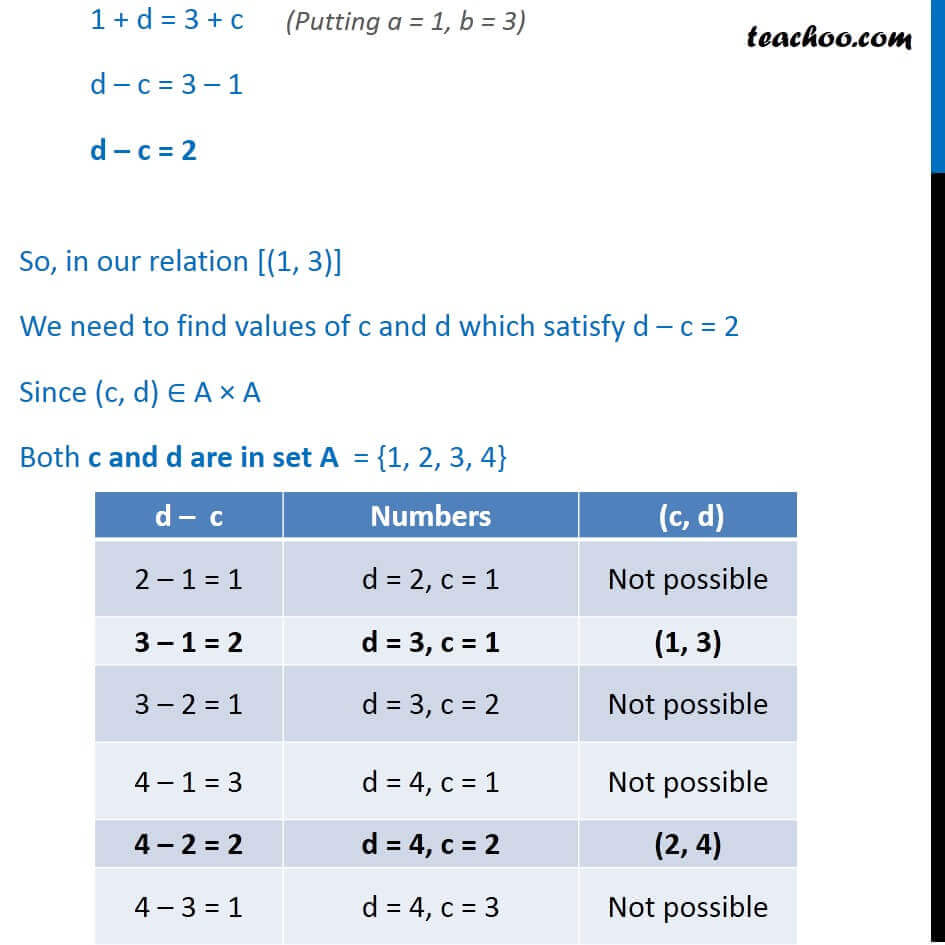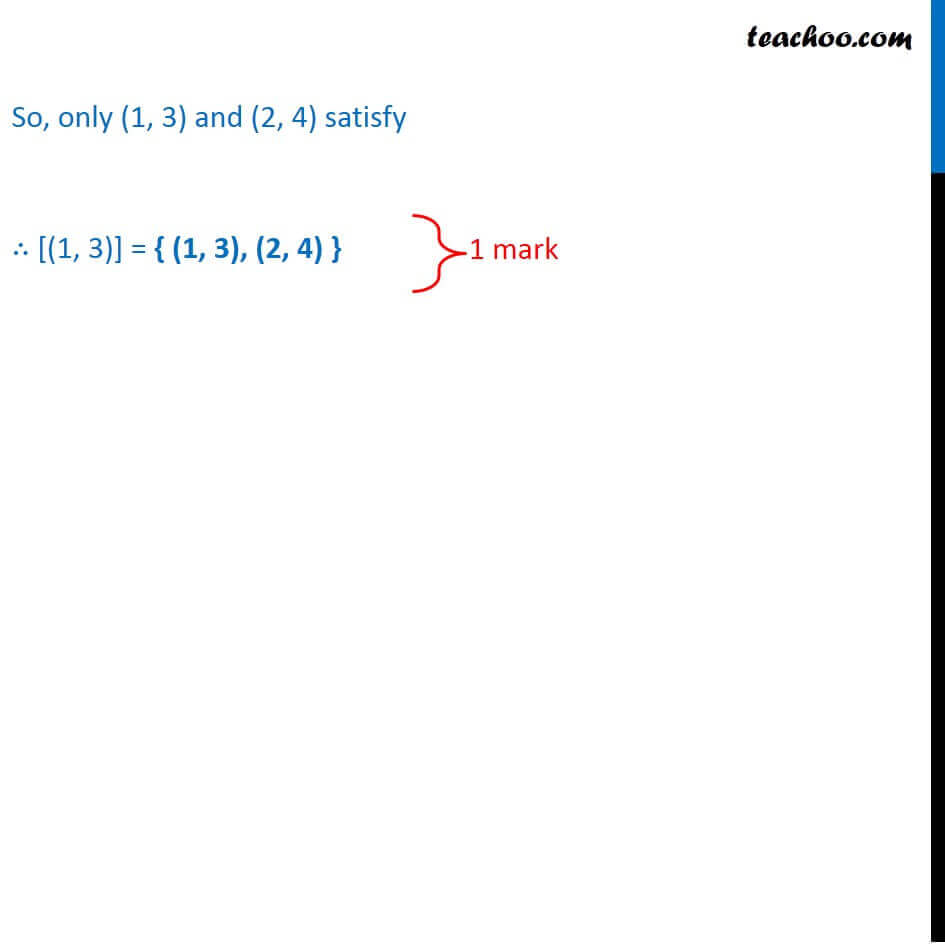CBSE Class 12 Sample Paper for 2018 Boards

Class 12
Solutions of Sample Papers and Past Year Papers - for Class 12 Boards

### Let A = {1, 2, 3, 4}. Let R be the equivalence relation on A × A defined by (a, b)R(c, d) iff a + d  = b + c . Find the equivalence class [(1, 3)].

This is a question of CBSE Sample Paper - Class 12 - 2017/18.Learn in your speed, with individual attention - Teachoo Maths 1-on-1 Class

### Transcript

Question 1 Let A ={1, 2, 3, 4}. Let R be the equivalence relation on A × A defined by (a, b)R(c, d) iff a + d = b + c . Find the equivalence class [(1, 3)]. Given A = {1, 2, 3, 4} R is defined as (a, b)R(c, d) iff a + d = b + c In this relation (a, b) goes in , and (c, d) comes out. We need to find [(1, 3)] So, (1, 3) will go in, and (c, d) will come out This will be possible if a + d = b + c 1 + d = 3 + c d – c = 3 – 1 d – c = 2 So, in our relation [(1, 3)] We need to find values of c and d which satisfy d – c = 2 Since (c, d) ∈ A × A Both c and d are in set A = {1, 2, 3, 4} d – c Numbers (c, d) 2 – 1 = 1 d = 2, c = 1 Not possible 3 – 1 = 2 d = 3, c = 1 (1, 3) 3 – 2 = 1 d = 3, c = 2 Not possible 4 – 1 = 3 d = 4, c = 1 Not possible 4 – 2 = 2 d = 4, c = 2 (2, 4) 4 – 3 = 1 d = 4, c = 3 Not possible So, only (1, 3) and (2, 4) satisfy ∴ [(1, 3)] = { (1, 3), (2, 4) }